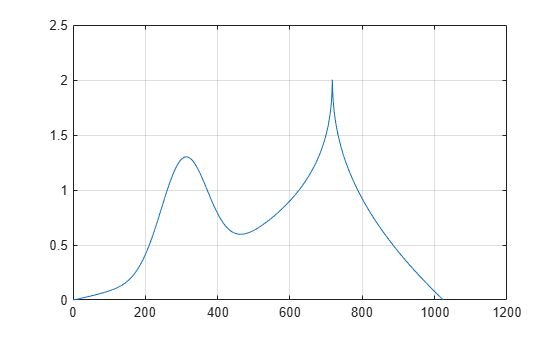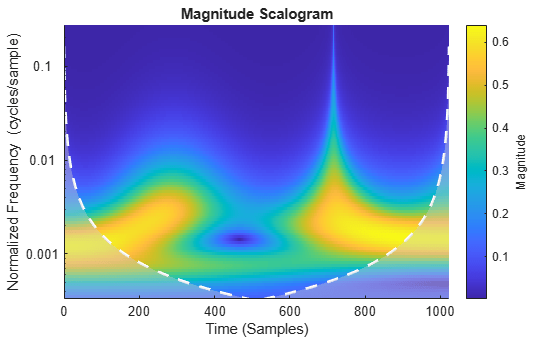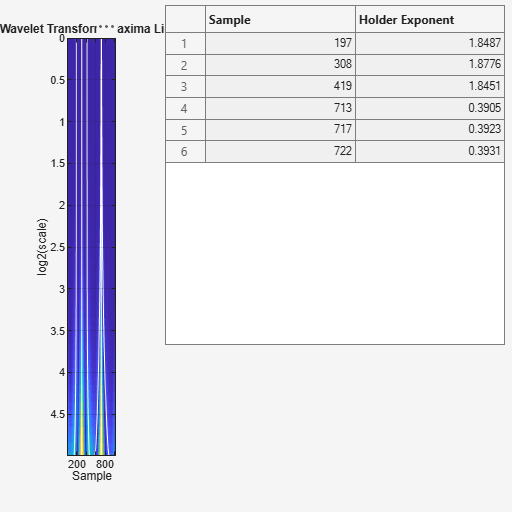# Continuous Wavelet Analysis of Cusp Signal

This example shows how to perform continuous wavelet analysis of a cusp signal. You can use `cwt` for analysis using an analytic wavelet and `wtmm` to isolate and characterized singularities.

Load and plot a cusp signal. Display its definition at the command line.

```load cuspamax; plot(cuspamax); grid on;````disp(caption)`
```x = linspace(0,1,1024); y = exp(-128*((x-0.3).^2))-3*(abs(x-0.7).^0.4); ```

Obtain and view the CWT of the cusp signal. The CWT uses an analytic Morse wavelet with gamma equal to 2 and a time-bandwidth parameter of 2.5. Notice the narrow region in the scalogram converging to the finest scale (highest frequency). This indicates a discontinuity in the signal.

`cwt(cuspamax,'WaveletParameters',[2 2.5]);`Obtain a plot of the wavelet maxima lines using wavelet transform modulus maxima. `wtmm` returns estimates of the Holder exponents, which characterize isolated singularities in a signal. Notice that the cusp is shown very clearly using `wtmm`.

`wtmm(cuspamax,'ScalingExponent','local');`##### SupportGet trial now